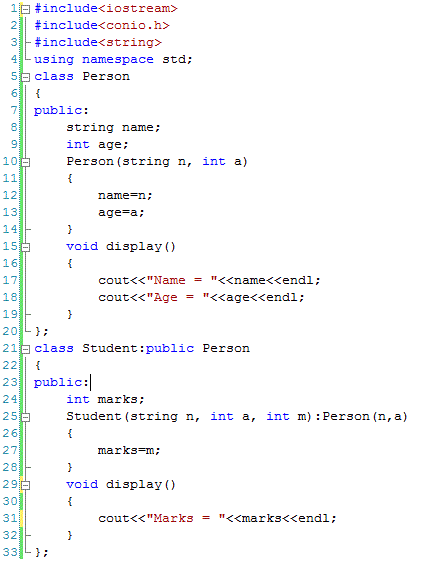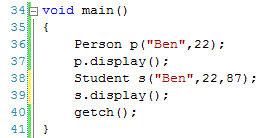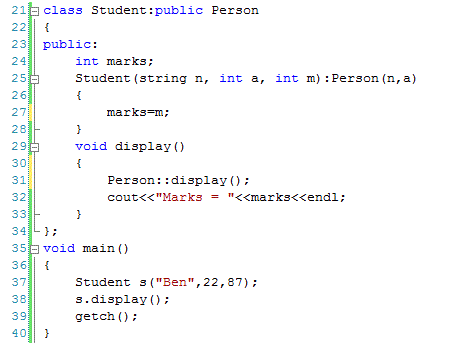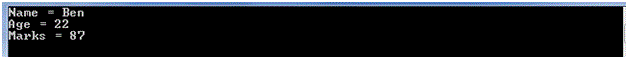# Function Overriding in C++

## Function Overriding in C++

Function overriding is a way to implement polymorphism. Polymorphism means to have more than one behaviors of same thing. If we have written a member function in the base class and the same member function is also written in the derived class having different implementation of the same member function then this is called function overloading. It means that inheritance is required for function overloading.

The following example shows function overriding where a member function display()is defined in the parent class Person and the same function is defined in the child class Student as well.After overriding, the member function can be called in a number of ways. For instance, we can create objects of class Person and of class Student. Each object will call the corresponding member function display as shown in the following code.We can see that display function is called at line 37 and 39. At line 37, the member function defined in class Person is called and at line 39, the member function defined in class Student is called.

How to Call Base Class Function

It may be required to call the overridden member function of parent class from the child class. For example when we call the display member function of child class then it should call the display member function of parent class first and then proceed with its own code.

In our case if an object of Student class call the display member function of Student class then first the display member function of Person class should be called to display the data member like name and age. Once name and age are displayed then the marks of the student should be displayed. This can be achieved as below.We can see that at line 31, the display member function of Student class calls the display member function of its parent class Person first and the will proceed with its own code. As a result if we create an object of Student class (line 37) and call the display member function of Student class then both the member functions written in the Person class and the Student class will be called. If we run the above code then the following output will be produced.It is important to note that the first two lines of the output come as a result of calling the display member function of Person class and the last line of the output comes from the display function written in the Student class.